Mobile QR Code1. (Department of Electronic Engineering, National Kaohsiung University of Science and Technology, Kaohsiung, Taiwan)

Terms—Transimpedance amplifier (TIA), optical receiver, high-speed transmission, four-level pulse amplitude modulation (PAM-4), complementary metal-oxide-semiconductor (CMOS)

## I. INTRODUCTION

With the development of high data throughput applications such as 4K video-on-demand, Internet of Things, and 5G mobile networks, high-speed fiber-optic transmission technology is an indispensable technology in data transmission systems (1). A transimpedance amplifier (TIA) is an important electrical device in the optical receiver of fiber-optic transmission,. Generally, the required bandwidth of a TIA is the data bit rate of the nonreturn to zero (NRZ) signal of 70%-80%. The design of a broadband TIA circuit becomes more difficult as the operating bit rate increases. Recently, a four-level pulse amplitude modulation (PAM-4) signal format has been widely used in 25-Gb/s applications (2,3). The data bit rate of the PAM-4 signal can be double that of the NRZ signal at the same operating bandwidth.

The circuit operating speed of an integrated circuit (IC), is most dependent on the process technology of the transistor. Presently, many broadband TIAs are realized in shorter-channel complementary metal-oxide-semiconductor (CMOS), SiGe, GaAs, or InP process technology (4,5); however, these process technologies are expensive. Broadband TIAs are faced with circuit design challenges when using a longer-channel CMOS process, so the bandwidth extension techniques should be included in a TIA circuit. Inductors are often used in broadband circuits to compensate for the effects of the parasitic capacitance and to extend the circuit bandwidth. However, in ICs, a planar spiral inductor is a large-area component that is difficult to control, so an inductorless broadband circuit has the advantage of reducing the chip area.

Because TIAs receive a current signal, the circuit requires low input impedance. TIAs with low input impedance can isolate the effect of the parasitic capacitance of the photodiode (PD). An equalizer is typically used to compensate for the high-frequency decay of the circuit. Therefore, in this study, we propose a broadband TIA circuit with low input impedance input stage and an equalization output stage in CMOS 90-nm technology. Our TIA was pre-set to be used at 25-Gb/s NRZ and 50-Gb/s PAM-4.

Because the TIA chip will be used in the optical receiver module, a TIA test integrating the TIA chip and a PD on a printed circuit board (PCB) is an effective TIA test architecture and can obtain circuit performance for actual application. However, a TIA test using a PD will require an optical signal source, and the test will be more complex and expensive. Therefore, in this study, we test our TIA chip on a PCB but do not use a PD. The performance of our TIA chip will be observed and verified through eye diagram measurements.

## II. CIRCUIT ARCHITECTURE AND ANALYSIS

A regulated cascode (RGC) circuit is used as the input stage of the TIA (6,7). The core amplifier is a two-stage fully differential amplifier with active feedback. The output stage is a differential amplifier as well as an equalizer. Fig. 1 shows the circuit schematic diagram of our TIA. Table 1 shows the device parameters.

Table 1. Device parameters in our TIA circuit

 Device Parameter Mp1, Mp2, Mp3, Mp4 W/L = 8 μm/0.1 μm M1, M3 W/L = 8 μm/0.1 μm M2, M4 W/L = 10 μm/0.1 μm Mp5, Mp6, Mp7, Mp8 W/L = 2 μm/0.1 μm M5, M6, M7, M8 W/L = 3 μm/0.1 μm M9, M10 W/L = 1.3 μm/0.1 μm M11, M12 W/L = 5 μm/0.1 μm RD1, RD2 50 Ω RSS 300 Ω CSS 0.1 pF

In the RGC circuit, p-MOSFET active loads are used to replace resistive loads, thereby reducing the chip area. The input resistance of the RGC circuit is approximately 1/[g$_{\mathrm{m1}}$(1+g$_{\mathrm{m2}}$r$_{\mathrm{op}}$)], where g$_{\mathrm{mi}}$ is the transconductance of the Mi transistor and r$_{\mathrm{op}}$ is the output resistance of the p-MOSFET. The RGC circuit has extremely low input impedance, making it suitable for use as a current input stage. Because of the low input impedance, the input pole frequency of the RGC circuit does not interfere with the bandwidth of the TIA.

Because the core amplifier is an active feedback TIA, the core amplifier must be noninverting to obtain negative feedback, and the two-stage fully differential amplifier is noninverting. The transimpedance gain of the core amplifier is inversely proportional to the transconductance of the feedback transistor. The core amplifier also uses active loads.

The output stage is a differential amplifier with a source degenerated resistor and capacitor as an equalizer. The transfer function of the output stage can be written as

##### (1)
$\mathrm{H}_{\mathrm{out}}(\mathrm{s})=\frac{\mathrm{g}_{\mathrm{m} 11} \mathrm{R}_{\mathrm{D}}}{1+\frac{\mathrm{g}_{\mathrm{m} 11} \mathrm{R}_{\mathrm{ss}}}{2}} \times \frac{1+\frac{\mathrm{s}}{\omega_{z}}}{\left(1+\frac{\mathrm{s}}{\omega_{\mathrm{p} 1}}\right)\left(1+\frac{\mathrm{s}}{\omega_{\mathrm{p} 2}}\right)}$

where $\omega_{\mathrm{z}}$ = 1/(R$_{\mathrm{SS}}$C$_{\mathrm{SS}}$), $\omega_{\mathrm{p1}}$ = 1/(R$_{\mathrm{D}}$C$_{\mathrm{L}}$), $\omega_{\mathrm{p2}}$ = (1+g$_{\mathrm{m9}}$R$_{\mathrm{SS}}$/2)/(R$_{\mathrm{SS}}$C$_{\mathrm{SS}}$), and C$_{\mathrm{L}}$ is the output capacitance of the TIA. The zero frequency can be adjusted to compensate for the TIA’s high-frequency response and to extend the bandwidth when selecting R$_{\mathrm{SS}}$ and C$_{\mathrm{SS}}$ values. The output impedance of the output stage uses a 50-Ω resistor to match the impedance of the oscilloscope. Because of low output impedance, the equalizer cannot provide a higher gain for TIA.

Fig. 2. Post-layout simulations of the TIA (a) DC transfer curve, (b) frequency response, (c) input referred current noise density, (d) total harmonic distortion.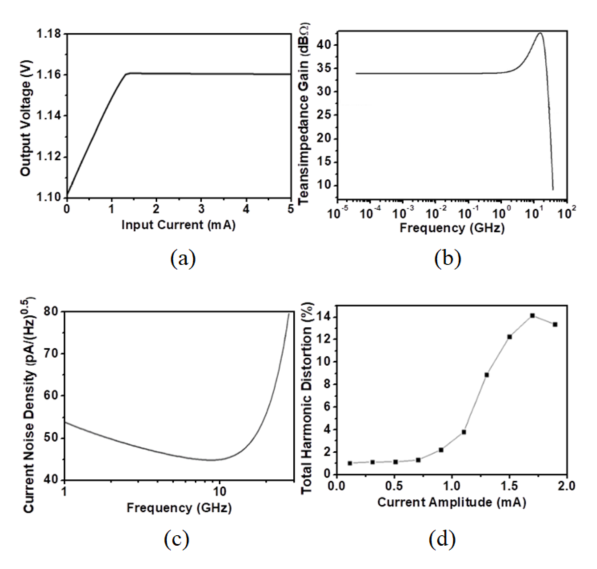Using TSMC 90-nm CMOS technology, we designed the TIA. We also completed the TIA’s circuit layout design and parasitic component extraction. The TIA chip size is 0.343 ${\times}$ 0.44 mm$^{2}$. The TIA performance was simulated and analyzed using the HSPICE simulator (8). According to direct current analysis, the input current range of TIA can be approximately 1.1 mA for linear operation when the output swing reaches 80% of the fully limited value, as shown in Fig. 2(a). In post-layout simulated frequency response, the PD equivalent circuit with a 0.3-F capacitor paralleling with a 2-kΩ resistor was used in the TIA. In Fig. 2(b), a 3-dB bandwidth of 24.8 GHz and a single-end transimpedance gain of 33.8 dBΩ at a low frequency are observed. The differential transimpedance gain was approximately 39.8 dBΩ. Therefore, the TIA can be operated at 25-Gb/s NRZ and 50-Gb/s PAM-4. An input-referred current noise density was also simulated, as shown in Fig. 2(c). The input referred-current noise density was less than 70 pA/${\sqrt{}}$Hz within a 3-dB bandwidth, and the average was estimated to be approximately 50 pA/${\sqrt{}}$Hz. Because active feedback generally tends to contain more noise than resistance feedback, the input-referred current noise density of the TIA seemed high, and it could result a high signal-to-noise ratio (SNR) of the output signal. We also simulated total harmonic distortion of the TIA, as shown in Fig. 2(d).

An average noise current of 7.87 μA$_{rms}$ was estimated from the current noise density, so the minimum input current amplitude was approximately 110 μA$_{pp}$ under a bit error rate of 10$^{{-}12}$ (9). The total harmonic distortion could be less than 5% when the input current amplitude was less than 1.1 mA$_{\mathrm{pp}}$. Thus, 25-Gb/s NRZ and 50-Gb/s PAM-4 output voltage eye diagrams were simulated when the amplitude of input current signals were the minimum input current of 110 μA$_{pp}$ and the maximum of 1.1 mA$_{\mathrm{pp}}$. Fig. 3 shows the post-layout simulated eye diagrams of the signal-end output voltage of the TIA. At the minimum input current, the 25-Gb/s NRZ and 50-Gb/s PAM-4 eye diagrams are clear, and the top, middle, and bottom eye-heights of the 50-Gb/s PAM-4 eye diagram are almost equal. At the maximum input current, the 25-Gb/s NRZ and 50-Gb/s PAM-4 eye diagrams are still clear, but a slight nonlinear distortion can be observed.

Fig. 3. Post-layout simulated output voltage eye diagrams of the TIA for (a) 25-Gb/s NRZ at 110-μA$_{pp}$ input current, (b) 25-Gb/s NRZ at 1.1-mApp input current, (c) 50-Gb/s PAM-4 at 110-μA$_{pp}$ input current, (d) 50-Gb/s PAM-4 at 1.1-mApp input current.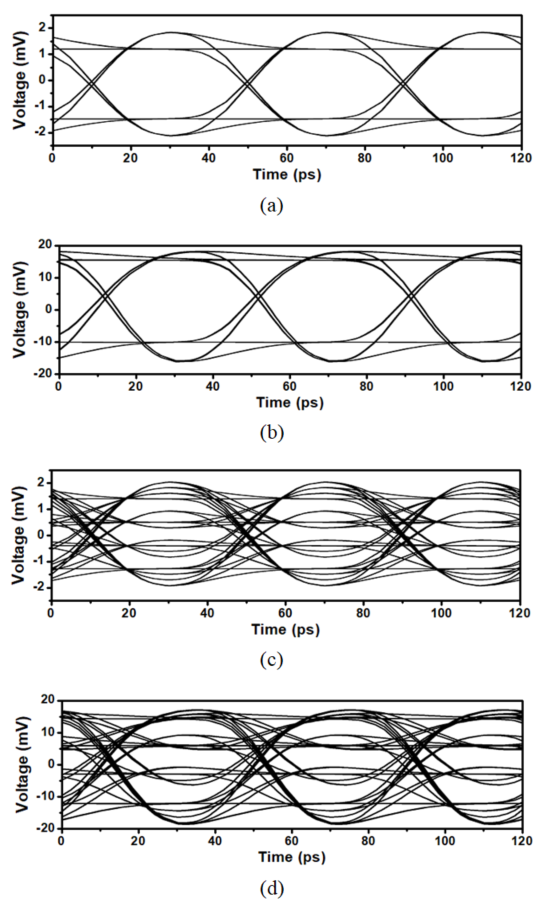Fig. 4. Measurement architecture for the TIA chip on a PCB.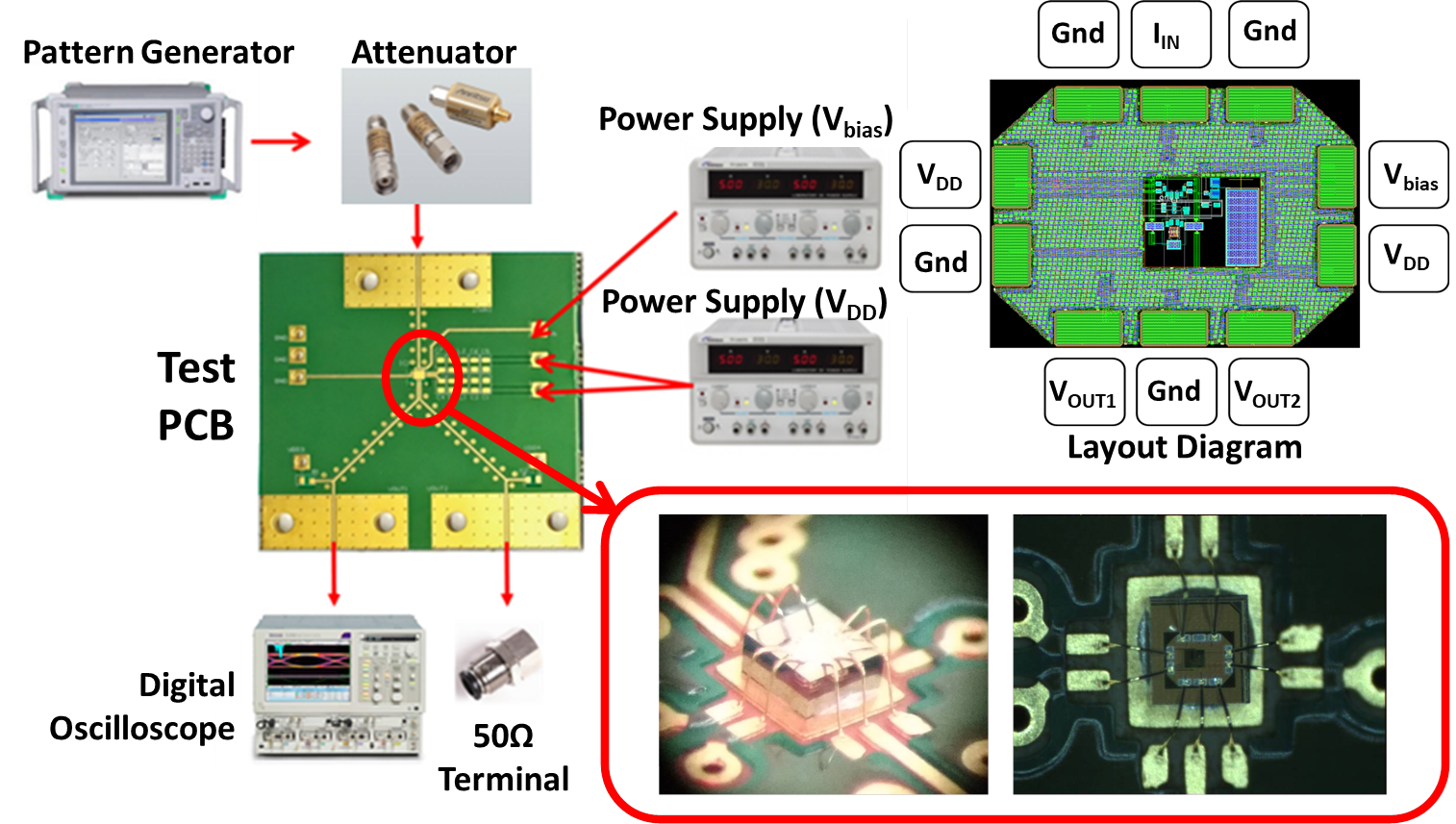## III. MEASURED VERIFICATION

The TIA chip was die- and wire-bonded on a PCB. The high-frequency PCB material is Rogers 4350B. The high-frequency signal transmission line on the PCB uses the grounded coplanar waveguide structure. The PD was not used in the TIA chip testing. Fig. 4 shows the measurement architecture of our TIA chip testing. The high-speed signal is provided from the pattern generator (Anritsu MP 1800A). Because the input signal requires a small current signal, a ${-}$20 dB attenuator is used to reduce the signal amplitude. The output voltage signal of the TIA can be observed through a high-speed digital oscilloscope (Tektronix DSA8300). The single-end output signal is measured and the other output end was connected with a 50-Ω terminal resistor.

When the ${-}$20-dB attenuator is not used, the 25 Gb/s NRZ electrical eye diagram from the pattern generator is shown in Fig. 5(a), the rise time of the eye diagram is 14.4 ps, the fall time is 13.6 ps, the time jitter is 2.6 ps$_{\mathrm{pp}}$, and the SNR is 31.6. Fig. 5(b) shows the output eye diagram with a rise time of 25.8 ps, a fall time of 23.2 ps, a time jitter of 12.6 ps$_{\mathrm{pp}}$, an SNR of 13.26, and an amplitude of 26 mV$_{\mathrm{pp}}$ when using the 25-Gb/s NRZ input signal with a current amplitude of 1.04 mA$_{\mathrm{pp}}$. The 25-Gb/s NRZ eye diagram is clear. The 40-Gb/s NRZ output eye diagram with a rise time of 19.3 ps, a fall time of 16.62 ps, a time jitter of 19.37 ps$_{\mathrm{pp}}$, an SNR of 4.45, an amplitude of 26 mV$_{\mathrm{pp}}$, and an eye height of 10 mV can be observed in Fig. 5(c). The 40 Gb/s NRZ eye diagram is also sufficiently clear.

Fig. 5. (a) 25-Gb/s NRZ eye diagram from the pattern generator, (b) 25-Gb/s NRZ eye diagram from the TIA output, (c) 40-Gb/s NRZ eye diagram from the TIA output.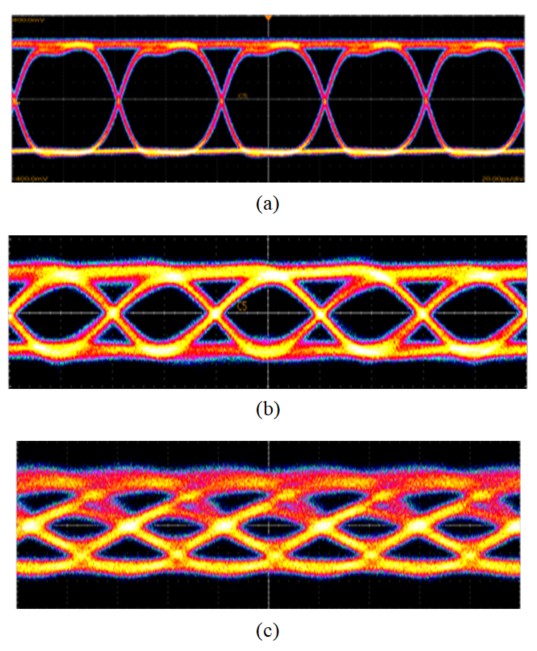From the pattern generator, the 50-Gb/s PAM-4 electrical eye diagram is shown in Fig. 6(a). The output eye diagram when using the 50-Gb/s PAM-4 input signal with a current amplitude of 1.36 mA$_{\mathrm{pp}}$ is shown in Fig. 6(b). The total output amplitude is approximately 28 mV, and the top, middle, and bottom eye heights are almost equal and approximately 3.5 mV. The TIA generally requires better SNR and larger output amplitude for the PAM-4 operation than for the NRZ operation. The transimpedance gain of our TIA can be improved. If the differential output signal eye diagrams could be measured, the SNR would be better.

Table 2 summarizes the performance of our TIA circuit. Comparison with related measurement works is also shown in Table 2. Because our TIA had a small chip area, low power consumption, and high bandwidth, it has a good figure of merit (FOM) value. However, the noise performance and transimpedance gain of our TIA chip should be improved.

Fig. 6. (a) 50-Gb/s PAM-4 eye diagram from the pattern generator, (b) 50-Gb/s PAM-4 eye diagram from the TIA output.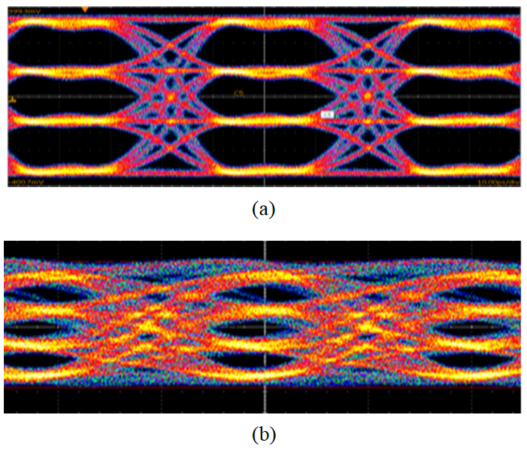Table 2. Comparison of the performance of some TIA circuits

 Ref.     This work CMOS Technology (nm) 90 65 65 55 90 Transimpedance Gain (dBΩ) 66 52.3 54 69 39.8 Bandwidth (GHz) 29 12.6 40 10.7 24.8 Data Bit Rate (Gb/s) 40 25 32 10 50 Input referred Current Noise (pA/$\mathrm{GHz}$) 22 18.1 19.8 15 50 Power Consumption (mW) 75 3.96 55.2 106 11.6 Chip Area (mm2) 0.15 0.63 0.6 0.21 0.15 FOM1 7.73 14.59 3.29 2.21 11.35 FOM2 10.67 28.96 2.64 2.07 22.87 \begin{aligned} & \text { FOM1 }=\frac{\operatorname{Gain}(\mathrm{dB} \Omega) \times \operatorname{Bandwidth}(\mathrm{GHz})}{\text { Noise }(\mathrm{pA} / \sqrt{H z}) \times \operatorname{Power}(\mathrm{mW}) \times \operatorname{Area}\left(\mathrm{mm}^{2}\right)} \\ & \text { FOM2 }=\frac{\operatorname{Gain}(\mathrm{dB} \Omega) \times \operatorname{Bit} \operatorname{rate}(\mathrm{Gb} / \mathrm{s})}{\text { Noise }(\mathrm{pA} / \sqrt{H z}) \times \operatorname{Power}(\mathrm{mW}) \times \operatorname{Area}\left(\mathrm{mm}^{2}\right)} \end{aligned}

## IV. CONCLUSIONS

The TIA with an RGC input stage, a core amplifier with active feedback, and an equalization output stage was implemented using TSMC 90-nm CMOS technology. The TIA has a wide bandwidth, good linearity, low power consumption, and a small chip size. According to eye diagram measurements, the TIA on a PCB has been verified and found to be capable of operating 25-Gb/s NRZ, 40-Gb/s NRZ, and 50-Gb/s PAM-4.

### ACKNOWLEDGMENTS

This work was supported in part by the Taiwan Semiconductor Research Institute and the Taiwan Ministry of Science and Technology, under the contract MOST 109-2224-E-992-001.

### REFERENCES

1
Cheng Q., Bahadori M., Glick M., Rumley S., Bergman K., 2018, Recent advances in optical technologies for data centers: a review, Optica, Vol. 5, No. 11, pp. 1354-13702
Bhoja S., March 2017, PAM4 signaling for intra–data center and data center to data center connectivity (DCI), in Opt. Fiber Commun. Conf., Los Angele, Vol. usa, pp. w4d.53
Isono H., January 2018, Latest standardization trends for client and networking optical transceivers and its future directions, in Proc. SPIE 10560, Metro Data Center Opt. Netw. Short-Reach Link, Vol. san francisco, pp. usa4
Knochenhauer C., Sedighi B., Ellinger F., 2011, 40 Gbit/s transimpedance amplifier with high linearity range in 0.13 μm SiGe BiCMOS, Electron. Lett., Vol. 47, No. 10, pp. 605-6065
Campbell C.F., Heins M.S., Kao M.Y., Muir M.E., Carroll J. M., June 2002, A 0.15-μm GaAs MHEMT transimpedance amplifier IC for 40-Gb/s applications, in IEEE MTT-S Int. Microwave Symp. Digest, Vol. seattle, No. usa, pp. 79-826
Park S.M., 2004, 1.25-Gb/s regulated cascode CMOS transimpedance amplifier for gigabit ethernet applications, IEEE J. Solid-State Circuits, Vol. 39, No. 1, pp. 112-1217
Seng C.W., Sern T.Y., Seng Y.K., August 2013, A low power wideband differential transimpedance amplifier for optical receivers in 0.18-μm CMOS, in IEEE 11th Int. New Circuit. Syst. Conf., Vol. paris, No. france, pp. 1-48
Hsu H.W., Peng C.C., Jou J.J., Shih TT T.T., Wu Y.D., Chu S.I., Lien C.Y., Liu B.H., July 2019, 25 Gb/s NRZ and 50 Gb/s PAM-4 transimpedance amplifier with active feedback and equalization in 90 nm CMOS technology, in 2019 Int. Conf. Opt. Commun Syst., Vol. prague, No. czech, pp. 251-2579
Sӓckinger E., 2005, Broad Circuits for Optical Fiber Communication, Ch. 4, Wiley, pp. 54-6610
Liao C.F., Liu S.I., June 2007, A 40Gb/s transimpedance-AGC amplifier with 19dB DR in 90nm CMOS, in IEEE Int. Solid-State Circuits Conf., San Francisco, Vol. usa, pp. 54-5511
Hiratsuka A., Tsuchiya A., Tanaka K., Fukuyama H., Miura N., Nosaka H., Onodera H., December 2018, A low input referred noise and low crosstalk noise 25 Gb/s transimpedance amplifier with inductor-less bandwidth compensation, in IEEE Asian Solid-State Circuits Conf., Vol. tainan, No. taiwan, pp. 69-7212
Kim S.G., Hong C., Eo Y. S., Kim J., Park S.M., 2018, A 40-GHz Mirrored-Cascode Differential Transimpedance Amplifier in 65-nm CMOS, IEEE J. Solid-State Circuits, Vol. 54, No. 5, pp. 1468-147413
Costanzo R., Bowers S.M., 2020, A 10-GHz bandwidth transimpedance amplifier with input DC photocurrent compensation loop, IEEE Microw. Wirel. Compon. Lett, Vol. 30, No. 7, pp. 673-676## Author

Jau-Ji Jou received B.S., M.S., and Ph. D. degrees from the Department of Electronic Engineering, National Taiwan University of Science and Technology, Taipei, Taiwan, in 1993, 1995, and 2002, respectively.

Since 2003, he has been with the Department of Electronic Engineering, National Kaohsiung University of Science and Technology, Kaohsiung, Taiwan, where he is currently a Professor.

His research interests include optical fiber communications, optical transmitter and receiver modules, and integrated circuit design for optical fiber communications.

Tien-Tsorng Shih received B.S. and Ph.D. degrees from the National Chiao Tung University, Taiwan, at 1986 and 1991, respectively.

In 1991, he joined Telecommunication Labo-ratories, Taiwan, as an associate researcher.

From 1996 to 2000, he was a project manager at Chunghwa Telecommunication Laboratories, Taiwan.

This team was a pioneer in developing communication laser diodes in Taiwan.

In 2000, he founded Infomax Optical Technology Corporation and was the CEO during 2000 to 2003.

After 2003, he becomes a faculty member of Department of Electronic Engineering, National Kaohsiung University of Science and Technology, Taiwan.

He has been a chair of the department during 2013−2016 and now is a distinguished professor and Dean of the College of Electrical Engineering and Computer Science.

His main research interests include high-speed circuit board design, optical transceiver, theoretical study of optical waveguides and III−V optoelectronic devices, silicon photonic device, high speed IC design, fabrication of laser diodes, photodiodes, packaging technology for optoelectronic devices, and transmission technologies for the fiber optics communication applications.

Chih-Chen Peng received an M.S. degree from the Department of Electronic Engineering, National Kaohsiung University of Science and Technology, Kaohsiung, Taiwan, in 2018.

His research interests include IC design for optical fiber communi-cations.

Hao-Wen Hsu received an M.S. degree from the Department of Electronic Engineering, National Kaohsiung University of Science and Technology, Kaohsiung, Taiwan, in 2020.

His research interests include IC design for optical fiber communi-cations.

Xuan-Yi Ye received a B.S. degree in 2020 and is currently pursuing the M.S. degree in the Department of Electronic Engineering, National Kaohsiung University of Science and Technology, Kaohsiung, Taiwan.

His research interests include IC design for optical fiber communications.